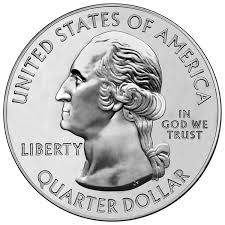Probability Level 5If you toss a fair coin 20 times, what is the probability of 5 successive heads at any time? Express the answer as a decimal and round it off to 7 decimal places.

This problem is not original. It is roughly as difficult as a problem in the BMO. This problem is part of this set.

×